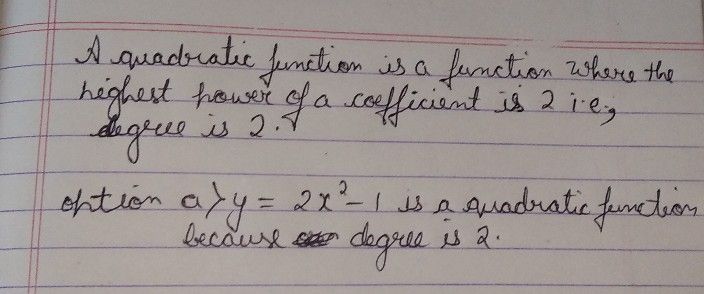Symbol
Problem$3$ 5. $-2$ $-1$ 1, $2$ $y$ $-1$ $\bar{1}$ $\bar{3}$ $\bar{57}$ $PART2$ $Di+00$ $1ons$ Choose the letter of the correct answer. VWrite your answer on the spac provided before each number. $1$ Which of the following equations represents a quadratic function? a $a.y=2x^{2}-1$ b. $3y=x+1$ $c.y=4x-52$ $d.y=6-2x$ $2.$ Determine which of the following equations define a quadratic function
1st-6th grade
Calculus
Search count: 107
SolutionQanda teacher - Animeshany doubt ask me